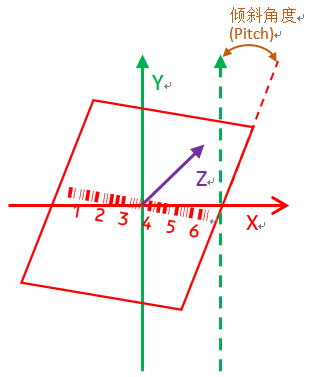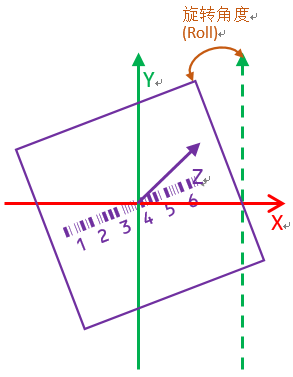# Welcome: NINGBO JIHAI IMPORT&EXPORT CO., LTD

Language: Español   English

# Introduce 3 angle parameters of the barcode scanner

When using a barcode scanner, we often see three angle parameters in the barcode scanner specification, which are Pitch, Roll, and Skew. What do these three angle parameters mean? Let's take a look at it.

Tilt angle refers to the angle between the plane and the Y axis when the bar code plane (the red plane in the figure below) rotates around the X axis.The rotation angle refers to the angle between the Y axis of the plane and the Y axis of the coordinate system when the bar code plane (the purple plane below) rotates around the Z axis.Deflection angle, the angle between the plane and the Y axis when the bar code plane (the green plane below) rotates around the Y axis## Categories

Contact: Colin

Phone: +86-15918668768

Tel: +86-15918668768